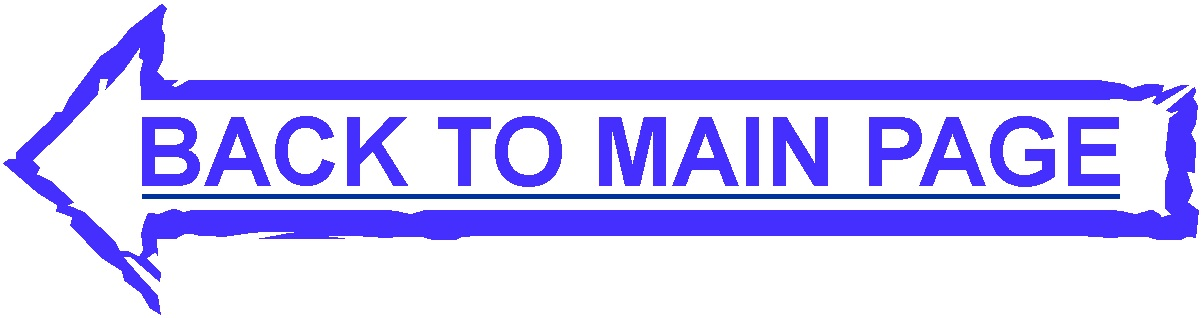Untitled Document

Learning Discrete Mathematics and Computer Science
via Primary Historical Sources

All Projects

Search Projects based on Courses

Sixteen projects developed before 2008 are posted at https://web.nmsu.edu/~davidp/hist_projects/

Projects developed since 2008 are listed below. Most of these projects have been published in Convergence. With each project there is a list of suggested courses where the project may be used and a list of topics covered in the project.

• Project 1: Sums of numerical powers in discrete mathematics: Archimedes sums squares in the sand 2010-10-07 Blog
Courses: Discrete Mathematics (lower division), Calculus
Topic: Summations, closed formulas, Riemann sums, areas, inequalities, telescoping sums, mathematical induction (optional), systems of linear equations (optional), recursive computation (optional)

• Project 2: Figurate numbers and sums of numerical powers: Fermat, Pascal, Bernoullii 2010-10-08 Blog
Courses: Combinatorics, Discrete mathematics (upper division)
Topic: sums of powers, figurate numbers, binomial coefficients, combination numbers, Pascal's triangle, Bernoulli numbers, recursive definitions and computation, summations and inequalities, counting and geometry, mathematical induction, interplay between discrete sums and calculus.

• Project 3: Deduction through the Ages: A History of Truth 2013-03-08 Blog
Courses: An introductory discrete mathematics course, A course in discrete mathematics for beginning computer science majors
Topic: Elementary propositional logic, truth tables, the truth table of an implication

• Project 4a: Origins of Boolean Algebra in the Logic of Classes: George Boole, John Venn and C. S. Peirce 2011-05-25 Blog
Courses: Discrete Mathematics (introductory)
Topic: elementary set operations (union, intersection, relative difference, symmetric difference) and their (algebraic) properties, Venn diagrams, relation of set operations to standard language use, relation of elementary set operations to boolean algebra, inverse operations (optional), issues related to mathematical notation (optional), changing standards of rigor and proof (optional)

• Project 4b: Boolean Algebra as an Abstract Structure: Edward V. Huntington and Axiomatization 2011-05-25 Blog
Courses: Discrete Mathematics (introductory or intermediate), Model Theory, Abstract Algebra
Topic: axioms for boolean algebra, boolean algebra properties (with proofs), two-valued model of boolean algebra, axiomatization, consistency, independence

• Project 4c: Applications of Boolean Algebra: Claude Shannon and Circuit Design 2011-05-25 Blog
Courses: Discrete Mathematics (introductory) for mathematics or computer science majors
Topic: boolean algebra axioms and properties, application of boolean algebra to design and simplification of circuits, simplification of boolean expressions, disjunctive normal form, two-valued model of boolean algebra

• Project 5: Euclid's Algorithm for the Greatest Common Divisor 2012-04-25 Blog
Courses: Discrete Mathematics (lower division), Introduction to Computer Science
Topic: Basic division properties of natural numbers, divisors and common divisors, greatest common divisor, basic reasoning, arguments and proofs, formal proof by induction (optional), basic algorithm design and formal description (pseudocode), reasoning about correctness of algorithms.

• Project 6: Algorithms, Recursion and Induction: Euclid and Fibonacci Blog
Courses: Discrete mathematics (sophomore level), Algorithms (junior/senior level)
Topic: Recursion and basic algorithm design using recursive thinking, comparing recursion with iteration, greatest common divisor, Fibonacci numbers, formal proof by induction, analysis of runtime and space complexity of algorithms including recursive algorithms, recurrence relations, dynamic programming.

• Project 7: Striving for efficiency in Algorithms: Sorting 2014-05-25 Blog
Courses: Data Structures and Algorithms
Topic: Sorting, quicksort, insertion sort, efficiency of algorithms, stack data structure.

• Project 8: Discovery of Huffman Codes 2014-05-22 Blog
Courses: Data Structures and Algorithms
Topic: Huffman encoding, greedy algorithm, prefix-free code, optimal code, unit of information, amount of information, binary tree

• Project 9: Networks and Spanning Trees 2013-06-18 Blog
Courses: Graph Theory, Combinatorics, Analysis of Algorithms
Topic: Graphs, Trees, Enumeration of labeled trees, minimal spanning trees, Cayley's formula and Prufer's symbols for labeled trees, Boruvka's algorithm for finding a minimial spanning tree.

• Project 10: Project on Program Correctness 2011-08-31 Blog
Courses: Algorithms, Data Structures, Programming Languages
Topic: Correctness, Partial Correctness, Algorithms

• Project 11: Abstract awakenings in algebra: Early group theory in the works of Lagrange, Cauchy, and Cayley 2011-05-25 Blog
Courses: Abstract Algebra
Topic: roots of unity, permutations, definition and elementary properties of a group, symmetric groups, cyclic groups, Cayley tables, Lagrange's Theorem, group isomorphisms, classification of groups of small order, cosets

• Project 12: Regular Languages and Finite Automata 2010-09-16 Blog
Courses: Automata and Formal Languages, Theory of Computation
Topic: Regular Languages, finite automata, regular expressions, regular events, Kleene's theorem, Kleene's star operation

• Project 13: Henkin's Method and the Completeness Theorem 2010-11-26 Blog
Topic: syntax of first-order logic, axioms and rules of inference, formal concept of proof, deduction theorem, satisfiability and validity, soundness, Henkin's method, completeness, Loewenheim-Skolem theorem, first-order logic with equality

• Project 14: Peano Arithmetic 2010-12-12 Blog
Topic: Peano postulates, consistency and independence of Peano postulates, recursive definition of addition and multiplication, properties of addition and multiplication, first-order arithmetic

• Project 15: Goedel's Incompleteness Theorems 2011-01-05 Blog
Topic: Self-referential statements and paradoxes, first-order languages and first-order theories, models, Peano arithmetic (PA), diagonalization lemma, incompleteness of PA (Goedel's first incompletenss theorem), unprovability of consistency (Goedel's second incompleteness theorem).

• Project 16: Undecidability of First-Order Logic Blog
Topic: Different formulations of the decision problem, models, isomorphic models, intended model, one-way infinite line, two-way infinite line, upper half-plane, Turing machines, halting problem, undecidability of first-order satisfiability

• Project 17: An Introduction to Elementary Set Theory 2011-09-20
Courses: Discrete/Finite Mathematics (sophomore/junior level)
Topic: Set, subset, set equality, union, intersection, set complement, empty set, powerset, Cartesian product, Russell's paradox, functions, images and inverse images, composition, one-to-one and onto functions, one-to-one correspondences and set equivalence, cardinal numbers, finite and infinite sets, countable and uncountable sets

• Project 18: Introduction to Symbolic Logic 2011-09-03 Blog
Courses: An introductory course in discrete mathematics, A course in discrete mathematics for beginning computer science majors
Topic: Propositional logic, logical connectives, logical equivalence, truth tables, tautologies, inference rules, predicate logic, individual variables and predicates, universal and existential quantifiers, logical equivalence of quantified statements, inference rules in predicate logic.

Note: If you would like to use any of the projects please let us know and we will e-mail you a .tex file of the project which you can modify to better suit your class needs.